Start typing, then use the up and down arrows to select an option from the list.# Weak Base Strong Acid Titrations 2

Jules Bruno
237views
2
So here we're dealing with calculations before the equivalence point. Now recall or before the equivalents point. That means they're weak. Species is greater in amount than our strong species in this case are weak based will be greater amount than our strong acid. Here we have 250 moles of our conjugate base, which is also our weak base. And let's say we have here 200 moles of the strong acid. Remember, in an I C F chart, we only care about three things the strong species, the weak acid and it's conjugal base. The fourth one we ignored here we have initially zero of our weak acid, but there are times that we could actually have something there. Remember, Look at the react inside. The smaller moles will subtract from the larger one. That gives us 0.50 moles of our contact base remaining zero bar strong acid. Here we have the addition of 0.200 moles at the end of this, what do we have left? We have weak acid remaining. We have conjugal based remaining. So we have a buffer that's left. So we have a weak acid left and conjugate base because we have a buffer. We can use the Henderson Hasselbach equation. So remember, that's just ph equals P K, which is the negative log of K plus log of conjugate base over weak acid. So that would be the negative log of what of r. K is of the weak acid plus log of our conjugate base. Over are weak acid. What? And that would help us get our pH. So again, before the equivalence point has been reached, our weak species is greater in amount. We still have a buffer, and therefore we can utilize the Henderson Hasselbach equation, click on to the next video and see what happens after the equivalence point.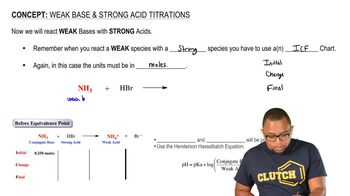01:49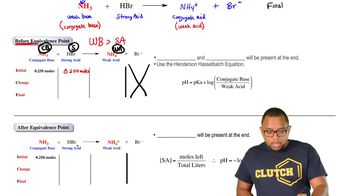02:15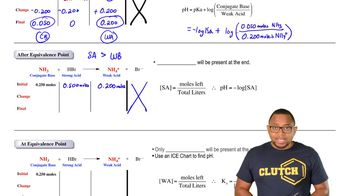01:39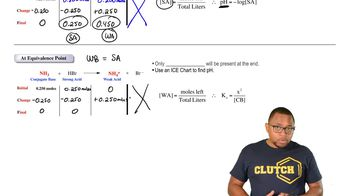01:41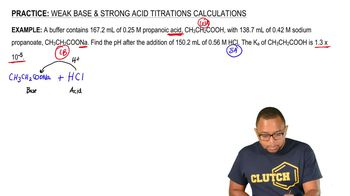05:37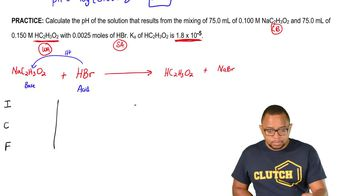04:19